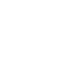Menu## What is the volumetric weight? And how do I calculate it?

Volumetric weight is a standard formula which is used in the airline industry and which includes density in the calculation of the transport costs. Volumetric weight is based on the volumetric standard of IATA (International Air Transport Association). On the basis of this calculation the space which the parcel will occupy inside the aircraft is compared with the actual weight of the parcel.

The shipping cost is based on the higher of the two values, in other words either the actual overall weight or the volumetric weight of the parcel. The higher weight is then used for the calculation of the shipping tariff.

The calculation of the volumetric weight depends on the individual service provider. As a rule the following formula is used:

Length x width x height / 6000 = volumetric weight

The DPD volumetric-weight calculator will help you to calculate the volumetric weight of your parcel quickly and conveniently.

(for shipments which contain a number of packages you simply add the individual results)